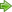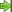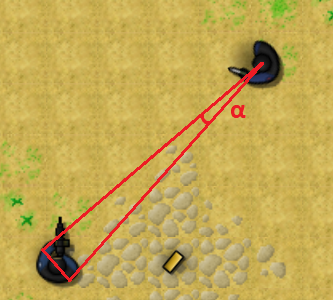﻿ Unreal Software - Thread: Check if two players are facing each other

# ForumCS2DScriptsCheck if two players are facing each other

#Check if two players are facing each other

26 replies
Goto Page1 2Mami Tomoe
User
OfflineHello, how can I check if two players are facing each other (I'm trying to override the shield logic with one of my own, but I got stuck at the part where I need to check for the angles).

All I need is some simple function like so:
Code:
1
2
3
4
5
6
7
8
local function RotationsFacingEachOther(rotA, rotB)
if <math stuffs> then

return true
end

return false
end

Of course, feel free to guide me to reaching the solution by myself, either way works!

Thanks.
Look at me standing, here on my own again
User
OfflineThat should be simple - it's basically if rotA = 360 - rotB (although you might want to use a margin here that gives you a wider range of angles the closer the opponent is).

You could also go all out Linear Algebra on this and assume the players viewing direction is a line and your opponent a circle and you check for collisions that way (and in reverse, too if you want both to be facing each other).
Mami Tomoe
User
OfflineWell, I tried this:

Code:
1
2
3
4
5
6
7
8
9
local function rotations_facing_each_other(rotA, rotB)
print(rotA, 360 -rotB)
if rotA == 360 -rotB then

return true
end

return false
end

And my output when doing this
Code:
1
lua print(rotations_facing_each_other(player(1,'rot'),player(2,'rot')))
in the console is this:
Code:
1
2
179.76782226563    357.00073242188
false

It seems to be too far off, even when we're pretty much looking at each other.
Look at me standing, here on my own again
Cebra
User
Offlinei suggest to look if the delta between the angles is 180.
In code, this means:
Code:
1
if math.abs(rotA-rotB) == 180 then ...

asHador suggested, i would use a margin, since it is really hard to get exactly 180 degree, for example like this:
Code:
1
if math.abs(rotA-rotB) <= 190 and math.abs(rotA-rotB) >= 170  then ...

edit: watch out, i noticed that player(id,"rot") return a value between -90 and 270. But - if i'm not wrong - it should work anyway. If this cause problems simply use
rotA % 360
Mami Tomoe
User
OfflineI tried the following:
Code:
1
2
3
4
5
6
7
8
9
10
function rotations_facing_each_other(rotA, rotB)
local abs = math.abs((rotA % 360) - (rotB % 360))

if abs <= 190 and abs >= 170 then

return true
end

return false
end

With and without the
% 360
part.
I don't seem to get consistent results.
When I look at the player holding the shield, it only sometimes works properly, and some other times, it reports
true
when it's supposed to be
false
.

Any idea on why that might be?
Look at me standing, here on my own again
Masea
Super User
Offline@Mami Tomoe: Print out
abs
to see whether that if statement even makes sense in the first place.
Mami Tomoe
User
Offline@Masea: In order to get the function to return
true
when I hit the shield I had to do this:
Code:
1
2
3
4
5
6
7
8
9
10
11
function rotations_facing_each_other(rotA, rotB)
local abs = math.abs((rotA % 360) - (rotB % 360))
hc.event(0,tostring(abs))

if abs >= 120 and abs <= 240 then

return true
end

return false
end

But, it still returns true even when I'm not pointing at the bot, I think it's hard to explain, someone might need to look into that.

Equip a bot with a Tactical Shield and just look at them and test to see if the function returns
true
when it's supposed to.
Look at me standing, here on my own again
ohaz
User
OfflineCalculate the angle of the line between the two players:
Quote:
angle1 = atan2(y2 - y1, x2 - x1)
angle2 = atan2(y1-y2, x1-x2)

And then check if angle1 is "close" to the view rotation of player1 and angle2 is close to the view rotation of player2
Code:
1
2
3
if abs(angle1-rot1) < 20 and abs(angle2-rot2) < 20:
return true
return false
https://ohaz.engineer - Software Engineering
Cebra
User
Offlinei've tested it now.
it does kinda work, but this part of the code only test, if the rotation of the players is "right".
Look here: https://prnt.sc/13mwhof
For your code both cases are the same.

edit:ohaz was fasterMami Tomoe
User
Offline@ohaz: This always returns
false
:
Code:
1
2
3
4
5
6
7
8
9
10
11
12
13
14
15
16
17
function rotations_facing_each_other(p, id)
local atan2     = math.atan2
local abs     = math.abs

local x1, y1 = player(p, 'x'),     player(p, 'y')
local x2, y2 = player(id, 'x'),     player(id, 'y')

local angle1 = atan2(y2 - y1, x2 - x1)
local angle2 = atan2(y1 - y2, x1 - x2)

if abs(angle1 - player(p, 'rot')) < 32 and abs(angle2 - player(id, 'rot')) < 32 then

return true
end

return false
end
Look at me standing, here on my own again
User
OfflineThe player-rotation (player(id,"rot")) is kinda scary. It goes from -90 to +270.

Use this script on a local server to see what I mean:
Code:
1
2
3
4
function yAy()
msg("Rotation: "..player(1,"rot"))
end

You wrote: atan(deltaY / deltaX) - player(p, 'rot') (in line 9, 11) which does NOT make sense. Your atan2 is in radiant while your rotation is in "degree"
edited 2×, last 31.05.21 09:14:50 pm
Share time limited free games hereMami Tomoe
User
Offline@Bowlinghead: Will using
on the two rotations help?

Also, should I always
rot % 360
my player rotations? Is it better? Should I write a wrapper for that?
Look at me standing, here on my own again
User
OfflineIm by no means an expert, but I would rather convert everything into degree for more precision and less perfomance costs.
Since I dont have further information: I assume your function runs on a timer, hence returns most of the time "FALSE".

Therefore I would split the directions the player is looking into 4 quadrants. Then I only have to deal with 0-90° and not with ugly negative numbers.

If one is looking top left and the other also top left, there is no point in doing heavy math.

Share time limited free games hereMami Tomoe
User
Offline@Bowlinghead:

It's used on the hit hook, to verify that a player is shooting onto another player's shield.
Look at me standing, here on my own again
User
OfflineI dont think that changes my argument, but I dunno. I just dont want to think about shotguns that shoot in a wide angle and you hit with the most outer bullet...What is the shield length by the way?
Share time limited free games hereGaios
Security Supporter
OfflineBowlinghead has written:
What is the shield length by the way?

The angle will be another depending on how close you're to the shield.

Code:
1
2
3
4
5
6
7
8
9
10
11
12
13
14
15
16
17
18
19
20
21
22
23
24
25
26
local atan2 = math.atan2
local deg = math.deg
local abs = math.abs

function point_direction(x1, y1, x2, y2)
return -deg(atan2(x1 - x2, y1 - y2))
end

function players_facing_each_other(p1, p2)
local angle = 12
local p1_rot, x1, y1 = player(p1, 'rot'), player(p1, 'x'), player(p1, 'y')
local p2_rot, x2, y2 = player(p2, 'rot'), player(p2, 'x'), player(p2, 'y')
p1_rot = (p1_rot < 0) and (p1_rot + 360) or p1_rot
p2_rot = (p2_rot < 0) and (p2_rot + 360) or p2_rot
local p1_dir = p1_rot - 180
local p2_dir = p2_rot - 180

local dir1 = point_direction(x2, y2, x1, y1)
local dir2 = point_direction(x1, y1, x2, y2)

if (abs(abs(dir1) - abs(p1_dir)) <= angle) and (abs(abs(dir2) - abs(p2_dir)) <= angle) then
return true
end

return false
end

Now what you need to do is to edit the
angle
, depending on how far the players are. Because the close players are, the wider angle is.I know how to calculate x1 y1 and x2 y2 of player width positions, but I have no idea how to deal with the 3 vectors and calculate the α (alpha).
edited 3×, last 01.06.21 12:01:05 am
SQ
Moderator
OfflineCalculating angles is more like a workaround.

This is how it should be solved properly using vectors.

Code:
1
2
3
4
5
6
7
8
9
10
11
12
13
14
15
16
17
18
19
20
21
22
23
24
25
26
27
28
29
30
31
32
33
34
35
36
37
38
39
40
41
42
43
44
45
46
47
48
49
50
51
52
53
54
55
56
57
58
59
60
61
62
63
64
65
66
67
68
69
70
71
72
73
74
75
76
77
78
79
80
81
82
83
84
85
86
87
88
89
90
91
function Dot(a, b)
return((a.x*b.x)+(a.y*b.y))
end

function Distance(a, b)
return math.sqrt((a.x-b.x)*(a.x-b.x)+(a.y-b.y)*(a.y-b.y))
end

function PointToLine(pixelPos, vA, vB)
local v0, v1 = {}, {}

v0.x = vB.x - vA.x
v0.y = vB.y - vA.y

local sqrt = math.sqrt(v0.x * v0.x + v0.y * v0.y)

v0.x = v0.x / sqrt
v0.y = v0.y / sqrt

v1.x = pixelPos.x - vA.x
v1.y = pixelPos.y - vA.y

local t = Dot(v0, v1)

if (t <= 0) then
return(vA)
elseif (t >= Distance(vA, vB)) then
return(vB)
end

local v2 = {}

v2.x = vA.x + v0.x * t
v2.y = vA.y + v0.y * t

return(v2)
end

function PlayersFaceEachOther(pid1, pid2)
if (player(pid1, "exists") == false or player(pid2, "exists") == false) then
return(false);
end

if (player(pid1, "health") < 1 or player(pid2, "health") < 1) then
return(false)
end

local p1, p2 = {}, {}
local p1raycast, p2raycast = {}, {}

-- needs check if player is in other player screen

-- player 1
p1.x = player(pid1, "x")
p1.y = player(pid1, "y")
p1.rot = math.rad(player(pid1, "rot") - 90)

p1raycast.x = p1.x + math.cos(p1.rot) * 320
p1raycast.y = p1.y + math.sin(p1.rot) * 320

-- player 2
p2.x = player(pid2, "x")
p2.y = player(pid2, "y")
p2.rot = math.rad(player(pid2, "rot") - 90)

-- raycast offsets
p2raycast.x = p2.x + math.cos(p2.rot) * 320
p2raycast.y = p2.y + math.sin(p2.rot) * 320

-- raycast p1
p1vec = PointToLine(p1, p2, p2raycast)
p2seeOther = Distance(p1vec, p1) < 16

-- raycast p2
p2vec = PointToLine(p2, p1, p1raycast)
p1seeOther = Distance(p2vec, p2) < 16

-- combine results
return(p1seeOther and p2seeOther)
end

function always()
seeEachOther = PlayersFaceEachOther(1, 2)

if (seeEachOther) then
parse("msg seeeachother *"..os.clock())
end

end

edited 7×, last 01.06.21 04:12:32 am
Mami Tomoe
User
Offline@SQ:
I'm going to assume I can just modify that always hook to be called whenever I need to check (which is on the hit hook).

I do have a question though, what does this mean:
Code:
1
-- needs check if player is in other player screen

Because in CS2D, there's a thing calledsv_offscreendamage, and depending on that, the check (if any was added) may or may not be needed.
Look at me standing, here on my own again
SQ
Moderator
OfflineI just left note that you might need to check if other player is offscreen in some scenarios.

Separated the logics from always hook.

Code:
1
2
3
4
5
6
7
8
9
10
11
12
13
14
15
16
17
18
19
20
21
22
23
24
25
26
27
28
29
30
31
32
33
34
35
36
37
38
39
40
41
42
43
44
45
46
47
48
49
50
51
52
53
54
55
56
57
58
59
60
61
62
63
64
65
66
67
68
69
70
71
72
73
74
75
76
77
78
79
80
81
82
83
84
85
86
87
88
89
90
function Dot(a, b)
return((a.x*b.x)+(a.y*b.y))
end

function Distance(a, b)
return math.sqrt((a.x-b.x)*(a.x-b.x)+(a.y-b.y)*(a.y-b.y))
end

function PointToLine(pixelPos, vA, vB)
local v0, v1 = {}, {}

v0.x = vB.x - vA.x
v0.y = vB.y - vA.y

local sqrt = math.sqrt(v0.x * v0.x + v0.y * v0.y)

v0.x = v0.x / sqrt
v0.y = v0.y / sqrt

v1.x = pixelPos.x - vA.x
v1.y = pixelPos.y - vA.y

local t = Dot(v0, v1)

if (t <= 0) then
return(vA)
elseif (t >= Distance(vA, vB)) then
return(vB)
end

local v2 = {}

v2.x = vA.x + v0.x * t
v2.y = vA.y + v0.y * t

return(v2)
end

function PlayersFaceEachOther(pid1, pid2)
if (player(pid1, "exists") == false or player(pid2, "exists") == false) then
return(false)
end

if (player(pid1, "health") < 1 or player(pid2, "health") < 1) then
return(false)
end

local p1, p2 = {}, {}
local p1raycast, p2raycast = {}, {}

-- needs check if player is in other player screen

-- player 1
p1.x = player(pid1, "x")
p1.y = player(pid1, "y")
p1.rot = math.rad(player(pid1, "rot") - 90)

-- player 2
p2.x = player(pid2, "x")
p2.y = player(pid2, "y")
p2.rot = math.rad(player(pid2, "rot") - 90)

-- raycast offsets
p1raycast.x = p1.x + math.cos(p1.rot) * 320
p1raycast.y = p1.y + math.sin(p1.rot) * 320

p2raycast.x = p2.x + math.cos(p2.rot) * 320
p2raycast.y = p2.y + math.sin(p2.rot) * 320

-- raycast p1
p1vec = PointToLine(p1, p2, p2raycast)
p2seeOther = Distance(p1vec, p1) < 16

-- raycast p2
p2vec = PointToLine(p2, p1, p1raycast)
p1seeOther = Distance(p2vec, p2) < 16

-- combine results
return(p1seeOther and p2seeOther)
end

function always()
seeEachOther = PlayersFaceEachOther(1, 2)

if (seeEachOther) then
parse("msg seeeachother *"..os.clock())
end
end

Offline@SQ: Thank you, I will test it out tomorrow!1 2